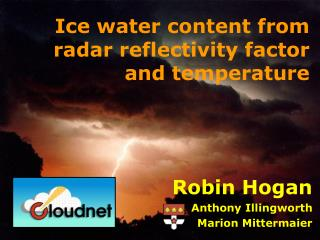DownloadDownload PresentationIce water content from radar reflectivity factor and temperature

# Ice water content from radar reflectivity factor and temperature

Télécharger la présentation## Ice water content from radar reflectivity factor and temperature

- - - - - - - - - - - - - - - - - - - - - - - - - - - E N D - - - - - - - - - - - - - - - - - - - - - - - - - - -
##### Presentation Transcript

1. Ice water content from radar reflectivity factorand temperature Robin Hogan Anthony Illingworth Marion Mittermaier

2. Overview • Use of mass-size relationships in calculating Z from aircraft size spectra in ice clouds • Radar-aircraft comparisons of Z • Derivation of IWC(Z,T): Rayleigh scattering • Evaluation of model IWC in precipitating cases using 3 GHz radar data • The problem of non-Rayleigh scattering • Derivation of IWC(Z,T): non-Rayleigh scattering

3. Interpretation of aircraft size spectra • To use aircraft size distributions to derive IWC(Z,T), need to be confident of mass-size relationship • Brown and Francis used m=0.0185D1.9 (SI units) • It produced the best agreement between IWC from size spectra and from independent bulk measurement • But can we use it for calculating radar reflectivity factor? • Use scanning 3 GHz data from Chilbolton during the Clouds, Water Vapour and Climate (CWVC) and Cloud Lidar and Radar Experiment (CLARE’98) • Rayleigh-scattering Z prop. to mass squared • Error in mass-size relationship of factor of 2 would lead to a 6 dB disagreement in radar-measured and aircraft-calculated values!

4. Comparisons from CLARE’98 T=-32ºC, Z=-0.7dB, m=-8% T=-15ºC, Z=-1.0dB, m=-11%

5. Comparisons from CWVC T=-21ºC, Z=+0.3dB, m=+3% T=-10ºC, Z=+0.3dB, m=+4%

6. Another CLARE case But this case was mixed-phase: liquid water leads to riming and depositional growth rather than aggregation: higher density T=-7ºC, Z=+3.7dB, m=+54% Implies particle mass/density is up to factor 2 too small

7. 3 GHz Mean slope: IWC~Z0.6

8. Relationship for Rayleigh scattering Observations by Field et al. (2004) demonstrate the T dependence of N0 • Relationship derived for Rayleigh-scattering radars: • log10(IWC) = 0.06Z – 0.0197T – 1.70 i.e. IWC  Z0.6f(T ) • What is the origin of the temperature relationship? • For an exponential distribution with density  D-1: • IWC  N0D03 and Z  N0D05 • If T is a proxy for D0 then eliminate N0: • IWC  ZD0-2  Zf(T ) • Not observed! • If T is a proxy for N0 then eliminate D0: • IWC  Z 0.6N00.4  Z0.6f(T ) • Correct!

9. IWC evaluation using 3 GHz radar • Now evaluate Met Office mesoscale model in raining events using Chilbolton 3 GHz radar • Advantages over cloud radar: • Rayleigh scattering: Z easier to interpret • Very low attenuation: retrievals possible above rain/melting ice • Radar calibration to 0.5 dB using Goddard et al. (1994) technique • Scanning capability allows representative sample of gridbox • 39 hours of data from 8 frontal events in 2000 • Apply IWC(Z,T) relationship and average data in horizontal scans to model grid • Threshold observations & model at 0.2 mm/h • Need to be aware of radar sensitivity; only use data closer than 36 km where minimum detectable reflectivity is –11 dBZ

10. Comparison of mean IWC • Results: • Accurate to 10% between –10ºC and -30ºC • Factor of 2 too low between -30ºC and -45ºC • Results at colder temperatures unreliable due to sensitivity sensitivity at 10 km sensitivity at 36 km

11. Comparison of IWC distribution • Distribution generally too narrow in model, problem worse at warmer temperatures

12. Non-Rayleigh scattering Mie-scattering using equivalent area diameter Mie-scattering using mean of max dimensions • Representation of Mie scattering has large effect… Equivalent-area diameter Mean of max dimensions Typical aircraft crystal image

13. 35 GHz log10(IWC) = 0.000242 ZT + 0.0699 Z – 0.0186T – 1.63 Non-Rayleigh scattering

14. 94 GHz log10(IWC) = 0.000580 ZT + 0.0923 Z – 0.00706T – 0.992 Non-Rayleigh scattering

15. Ice water Observations Met Office Mesoscale Model ECMWF Global Model Meteo-France ARPEGE Model KNMI RACMO Model Swedish RCA Model

16. Comparison of the IWC products (lidar/radar vs. Z,T) retrieved from Chilbolton data (2003) IWCZT =IWC Linear regression The linear regression fit in log-space of all data is close to the 1 to 1 line. The distribution is wide and not symmetric